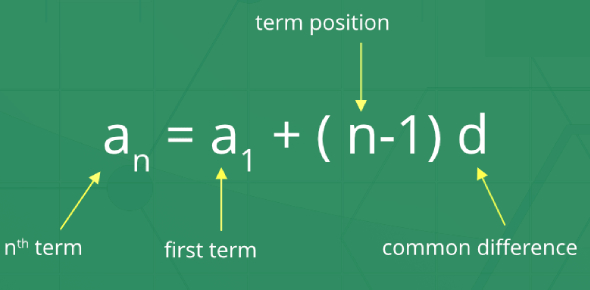# The Arithmetic Progression Test! Math Quiz

15 Questions | Attempts: 11943SettingsAre you ready for an arithmetic progression in the math quiz? Arithmetic progression math problems can be challenging for a person who does not know how to find the similarity between units in a sequence. The rule to understanding this problem is checking what the difference is from one number to another in a sequence. To help see how you know this concept, we have prepared the quiz below. Do give it a try and polish your skills.

• 1.
The common difference of the A.P. whose general term an = 2n + 1 is
• A.

-1

• B.

-2

• C.

2

• D.

1

• 2.
The number of terms in the A.P. 2, 5, 8,….., 59 is
• A.

20

• B.

19

• C.

12

• D.

25

• 3.
The first positive term of the A.P. -11, -8, -5,…..,
• A.

-4

• B.

3

• C.

-2

• D.

1

• 4.
The 4th term from the end of the A.P ….., 2, 5, 8,….., 35 is
• A.

20

• B.

29

• C.

23

• D.

26

• 5.
The 11th and 13th terms of an A.P. are 35 and 41 respectively its common difference is
• A.

6

• B.

3

• C.

38

• D.

32

• 6.
The next term of the A.P. ………. is
• A.
• B.
• C.
• D.
• 7.
Which of the following is not an A.P.?
• A.

-5, -2, 1, 4

• B.

11, 14, 17, 20

• C.

1, 4, 7

• D.

3, 7, 12, 18

• 8.
The sum of first 20 odd natural number is
• A.

400

• B.

285

• C.

421

• D.

281

• 9.
The sum of first 20 natural numbers is
• A.

210

• B.

190

• C.

170

• D.

110

• 10.
The sum of first 10 multiples of 7 is
• A.

385

• B.

406

• C.

315

• D.

371

• 11.
If the sum of the A.P. 3, 7, 11,………. is 210, the number is terms is
• A.

12

• B.

22

• C.

15

• D.

10

• 12.
If the sum of a1 + a10 = 50 for an A.P. Find the value of a1 + a2 +…… + a10?
• A.

150

• B.

200

• C.

250

• D.

300

• 13.
What would be the first negative term for an A. P. 121, 117, 113…..?
• A.

31

• B.

30

• C.

33

• D.

32

• 14.
The multiples of 4 that between 10 and 250?
• A.

51

• B.

61

• C.

71

• D.

81

• 15.
For an A.P. 9, 17, 25….the number terms inorder to give a sum of 636 is:
• A.

12

• B.

11

• C.

10

• D.

13

## Related TopicsBack to top
×

Wait!
Here's an interesting quiz for you.